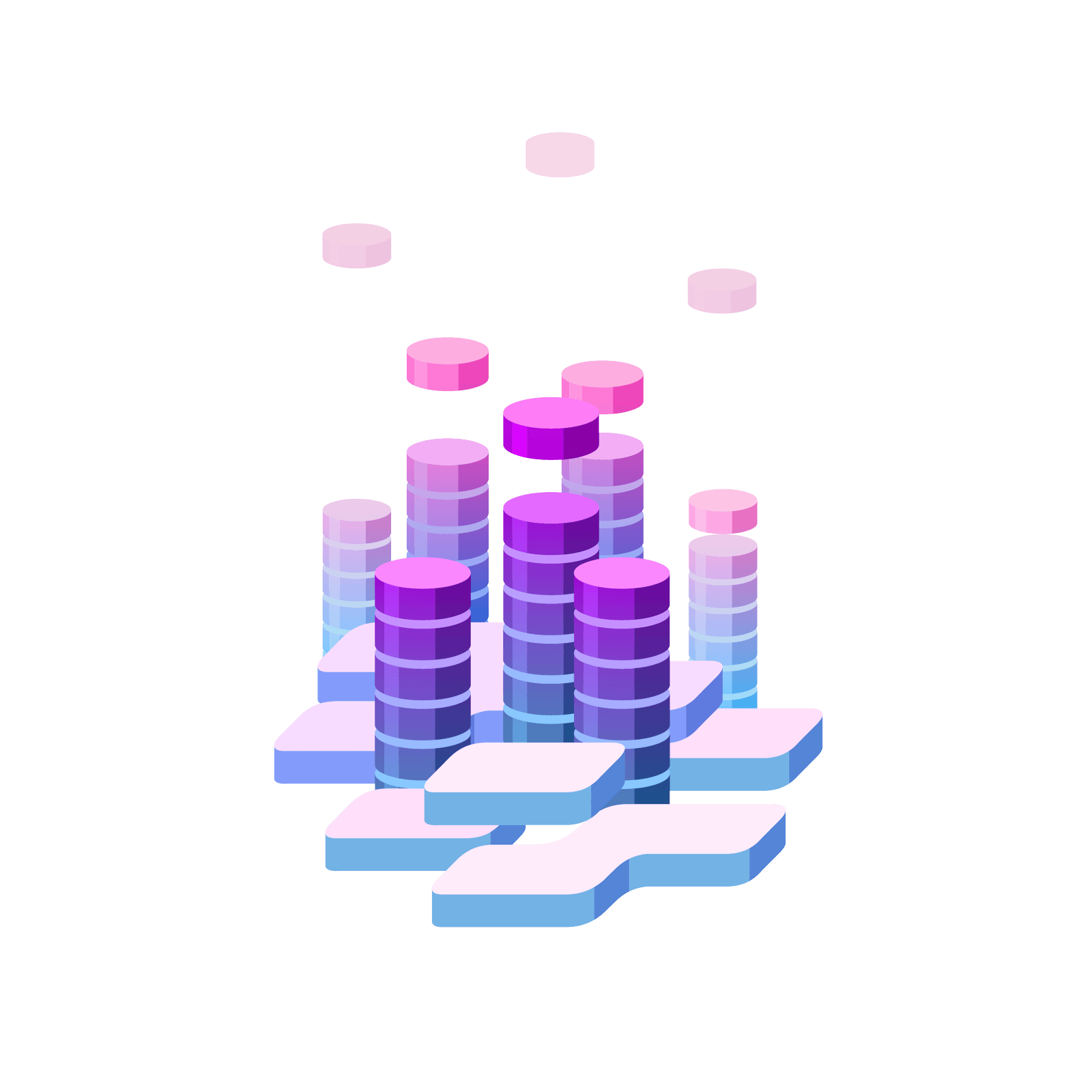06 285 000# Station  IWild ExplorationHere are some “big question” ideas you might want to explore, or just think about.
Have fun!

### Question 1

Here is an addition problem in a $1\leftarrow 5$ machine. (That is, it is a problem in base five.) This is not a $1\leftarrow 10$ machine addition.

What number has code $20413$ in a $1\leftarrow 5$ machine?
What number has code $13244$ in a $1\leftarrow 5$ machine?What is the $1\leftarrow 5$ machine answer?### THERE IS NOTHING SPECIAL ABOUT BASE TEN FOR MULTIPLICATION

Let’s now work with a $1\leftarrow 3$ machine.

Find $1202\times 3$ as a base three problem.

Also, what are $111\times 3$ and $2002\times 3$?

Can you explain what you notice?

(In base three we should really be writing $10$ rather than $3$ in each of these problems.)### Question 3

Let’s now work with a $1\leftarrow 4$ machine.

What is $133\times 4$?
What is $2011\times 4$?
What is $22\times 4$?

Can you explain what you notice?

(In base four we should really be writing $10$ rather than $4$ in each of these problems.)In general, if we are in a $1 \leftarrow b$ machine, can you explain why multiplying a number in base $b$ by $b$ returns the original number with a zero tacked on to its right?

### Let's Go Wild!